# Act III Function the Ultimate function expression function

• Slides: 73Act III Function the Ultimate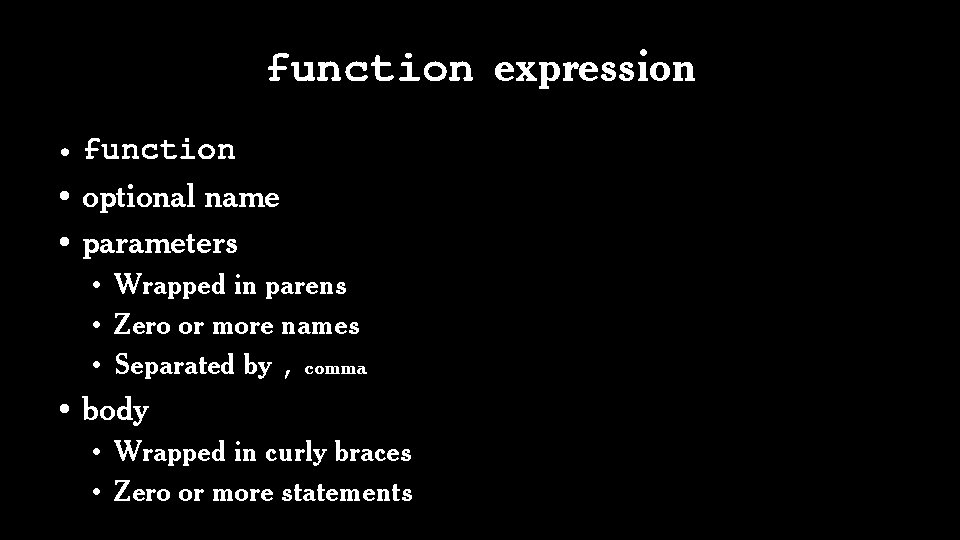function expression • function • optional name • parameters • Wrapped in parens • Zero or more names • Separated by , comma • body • Wrapped in curly braces • Zero or more statementsfunction expression • Produces an instance of a function object. • Function objects are first class. • • May be passed as an argument to a function May be returned from a function May assigned to a variable May be stored in an object or array • Function objects inherit from Function. prototype.function statement • function • mandatory name • parameters • Wrapped in parens • Zero or more names • Separated by , comma • body • Wrapped in curly braces • Zero or more statementsfunction statement • The function statement is a short-hand for a var statement with a function value. function foo() {} expands to var foo = function foo() {}; which further expands to var foo = undefined; foo = function foo() {}; It all gets hoisted to the top of the function.function expression v function statement If the first token in a statement is function , then it is a function statement.Scope A variable declared in a block is not visible outside of the block.Variables • const statement makes variables in block. Prohibits assignment to the variable. Not in IE. • let statement makes variables in block. Not in IE. • var statement makes variables in functions. Use var if it must run in IE. • Do not use both var and let.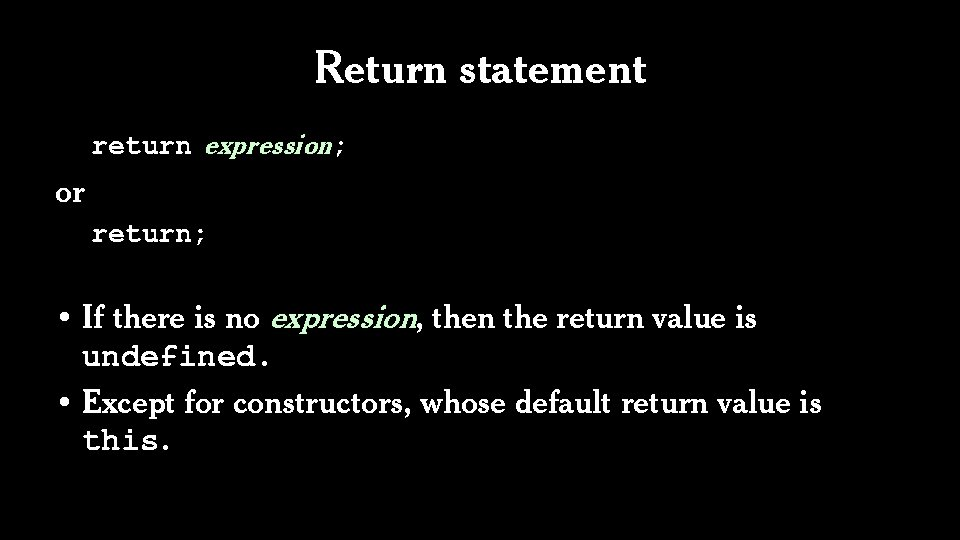Return statement return expression; or return; • If there is no expression, then the return value is undefined. • Except for constructors, whose default return value is this.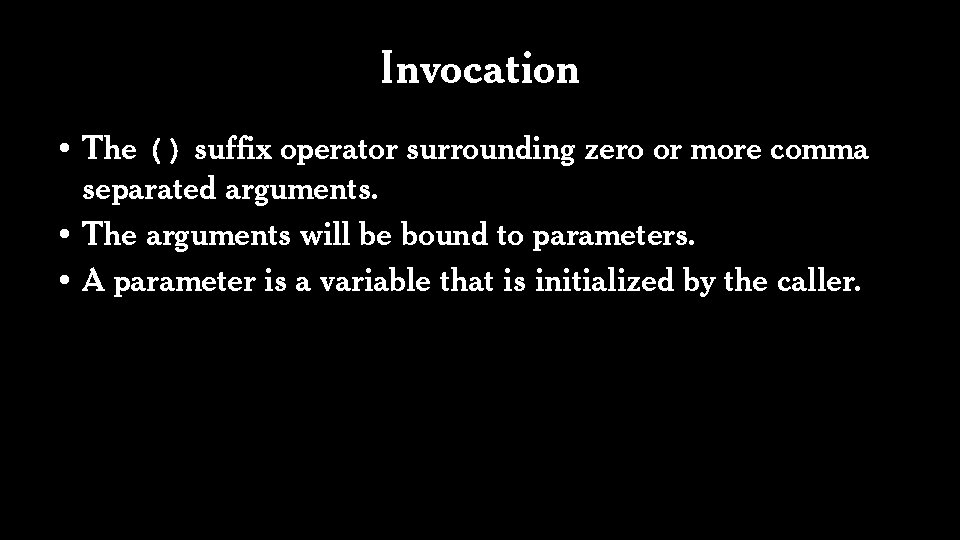Invocation • The () suffix operator surrounding zero or more comma separated arguments. • The arguments will be bound to parameters. • A parameter is a variable that is initialized by the caller.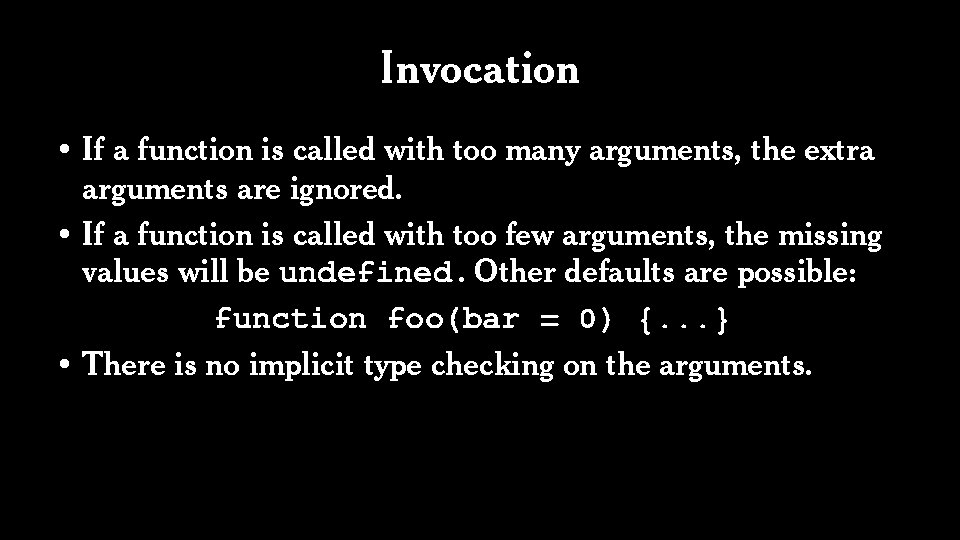Invocation • If a function is called with too many arguments, the extra arguments are ignored. • If a function is called with too few arguments, the missing values will be undefined. Other defaults are possible: function foo(bar = 0) {. . . } • There is no implicit type checking on the arguments.• Variable number of arguments: . . . • All remaining arguments are assembled into an array. function add(a, b) { return a + b; } function sum(. . . numbers) { return numbers. reduce(add); } var ten = sum(1, 2, 3, 4);Two pseudo parameters arguments this Neither is recommended.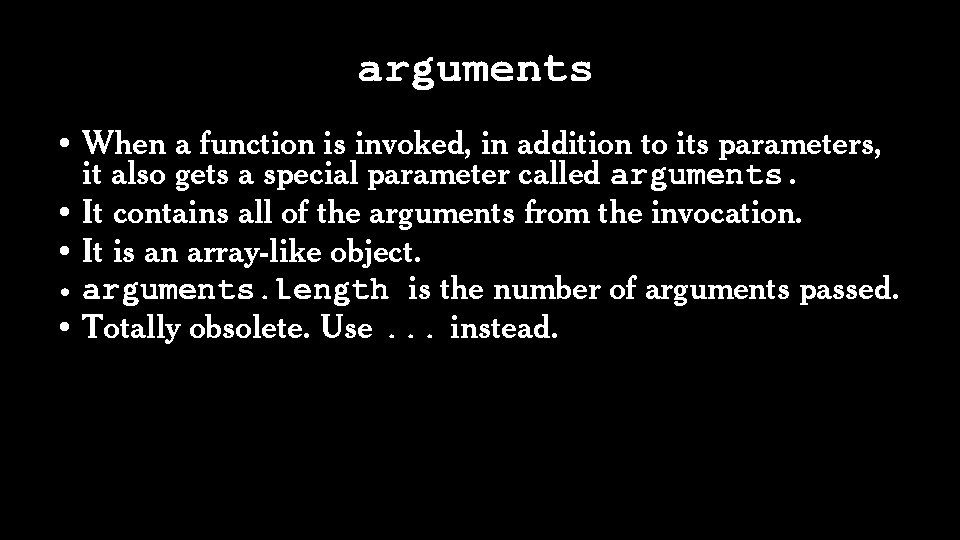arguments • When a function is invoked, in addition to its parameters, it also gets a special parameter called arguments. • It contains all of the arguments from the invocation. • It is an array-like object. • arguments. length is the number of arguments passed. • Totally obsolete. Use. . . instead.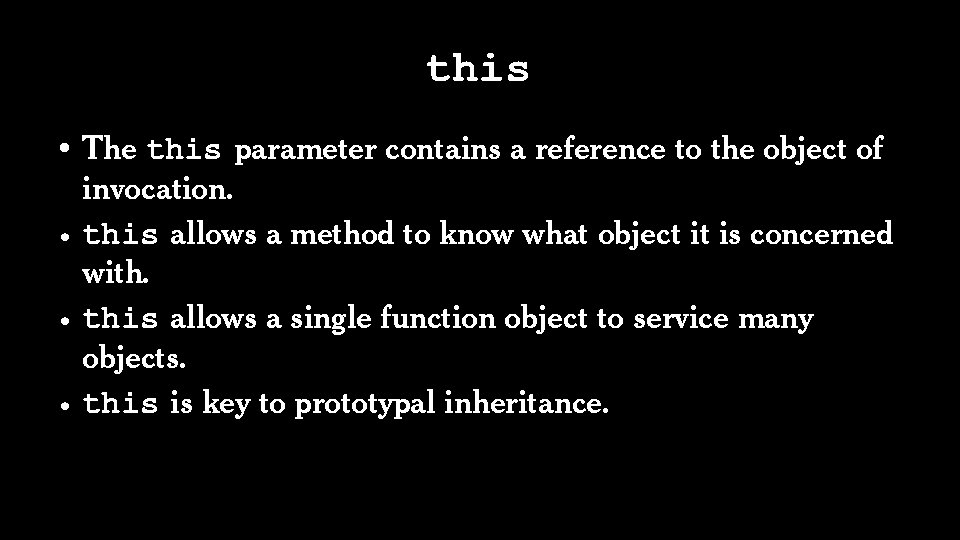this • The this parameter contains a reference to the object of invocation. • this allows a method to know what object it is concerned with. • this allows a single function object to service many objects. • this is key to prototypal inheritance.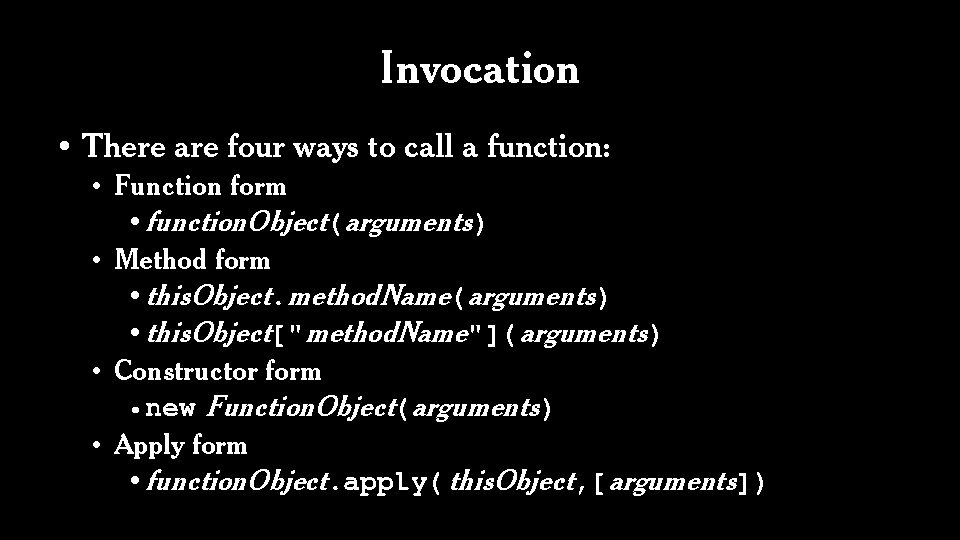Invocation • There are four ways to call a function: • Function form • function. Object(arguments) • Method form • this. Object. method. Name(arguments) • this. Object[" method. Name"]( arguments) • Constructor form • new Function. Object(arguments) • Apply form • function. Object. apply( this. Object, [ arguments])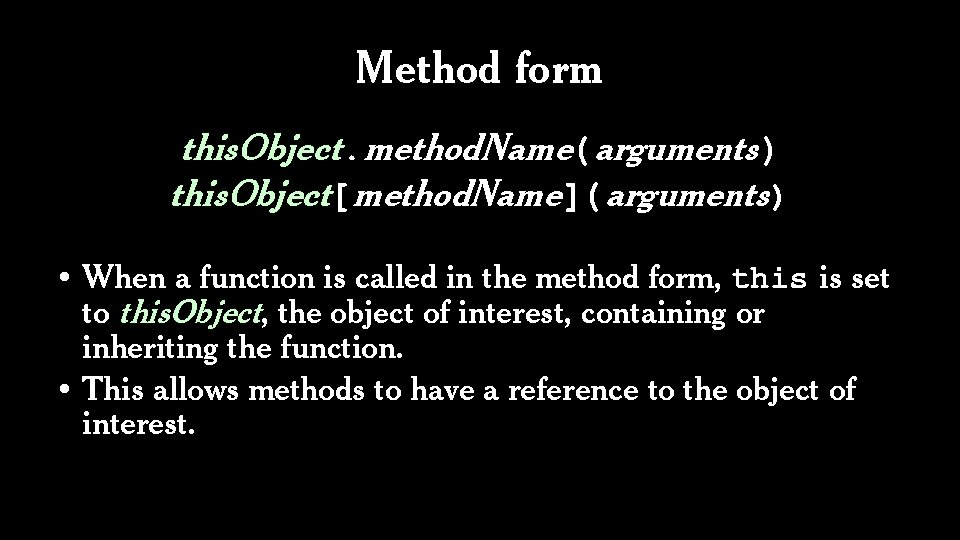Method form this. Object. method. Name(arguments) this. Object[method. Name]( arguments) • When a function is called in the method form, this is set to this. Object, the object of interest, containing or inheriting the function. • This allows methods to have a reference to the object of interest.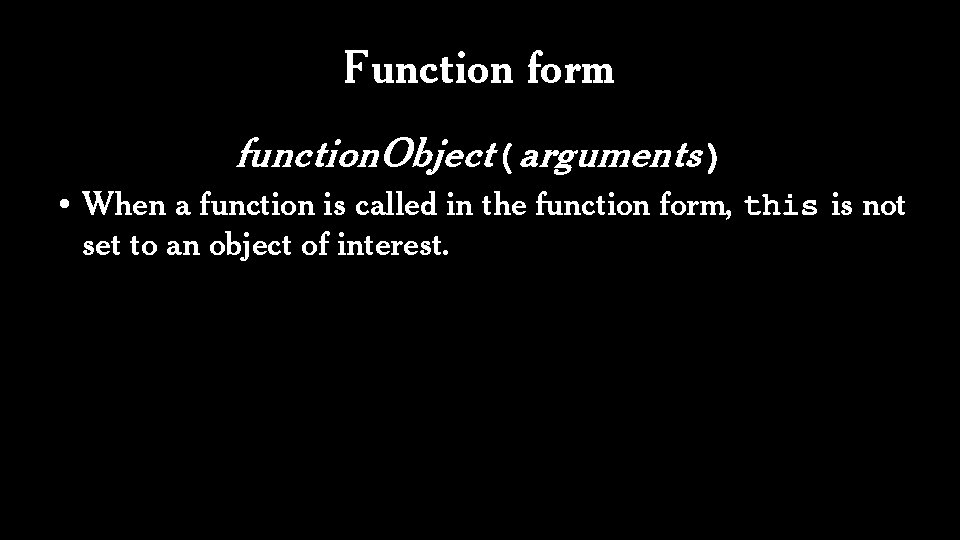Function form function. Object(arguments) • When a function is called in the function form, this is not set to an object of interest.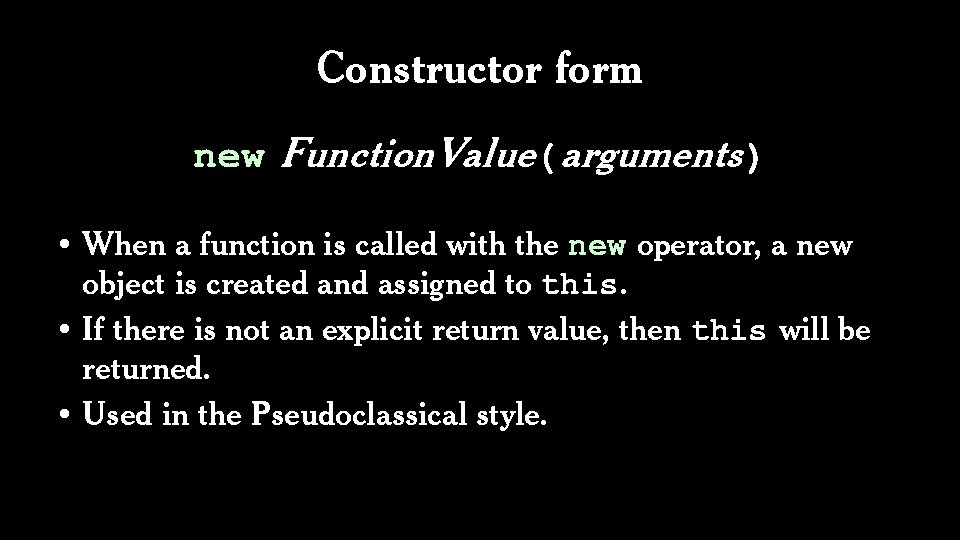Constructor form new Function. Value(arguments) • When a function is called with the new operator, a new object is created and assigned to this. • If there is not an explicit return value, then this will be returned. • Used in the Pseudoclassical style.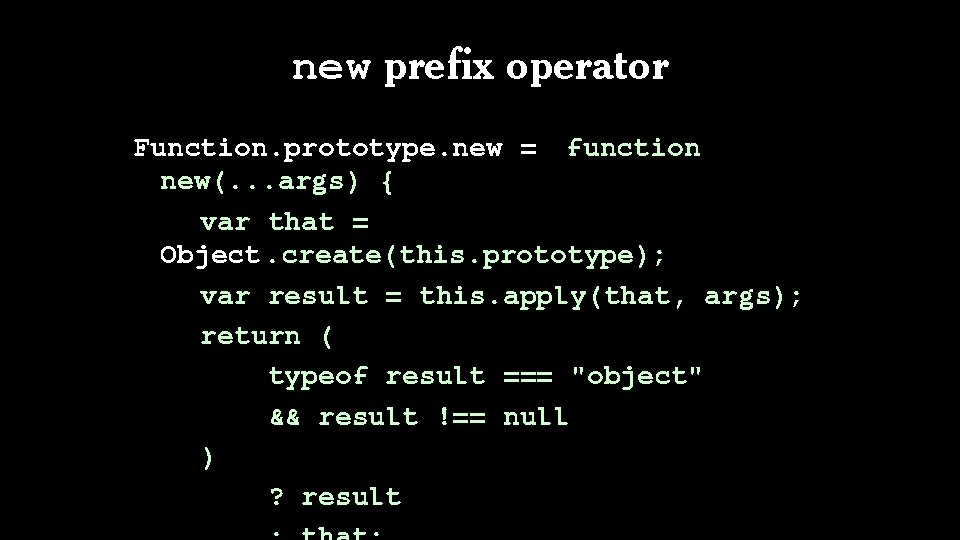new prefix operator Function. prototype. new = function new(. . . args) { var that = Object. create(this. prototype); var result = this. apply(that, args); return ( typeof result === "object" && result !== null ) ? result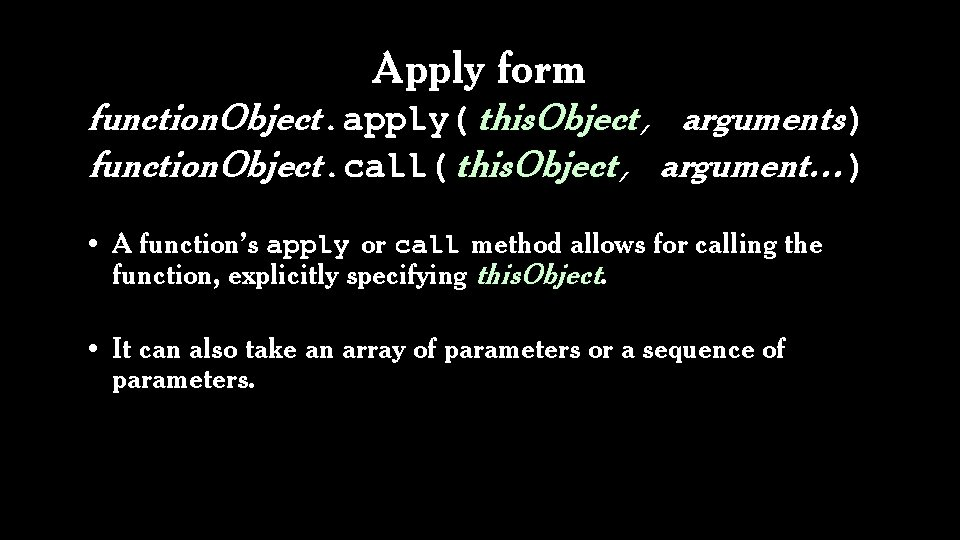Apply form function. Object. apply( this. Object, arguments) function. Object. call( this. Object, argument…) • A function’s apply or call method allows for calling the function, explicitly specifying this. Object. • It can also take an array of parameters or a sequence of parameters.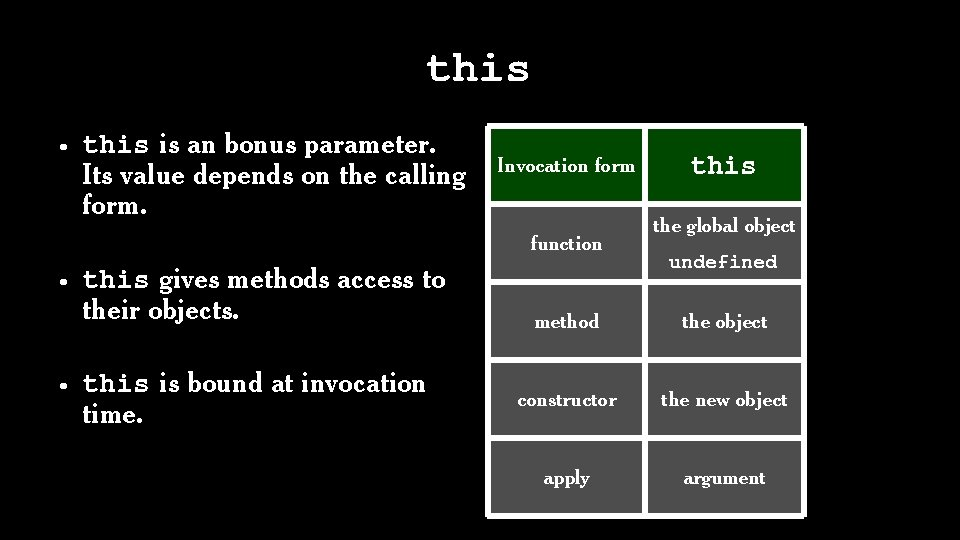this • this is an bonus parameter. Its value depends on the calling form. Invocation form function • this gives methods access to their objects. • this is bound at invocation time. this the global object undefined method the object constructor the new object apply argument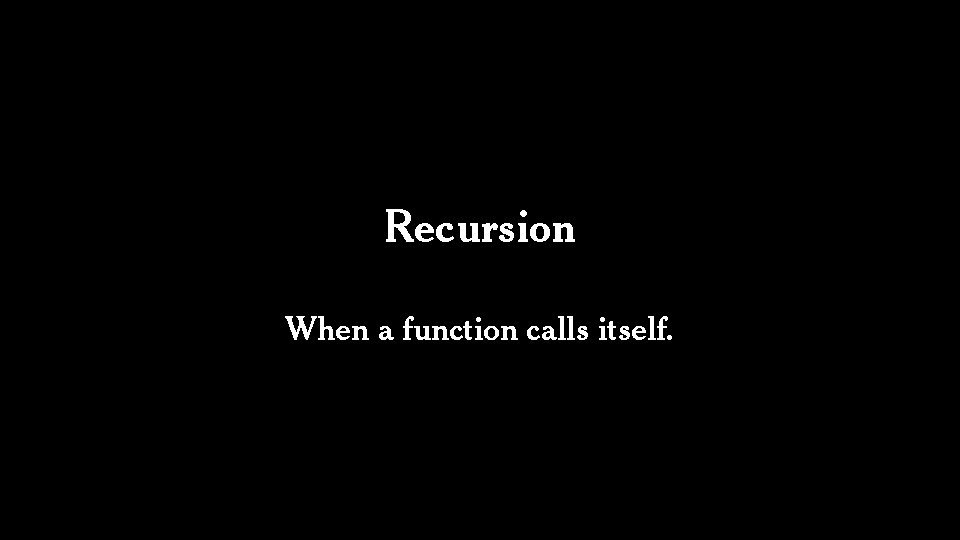Recursion When a function calls itself.The Little Lisper http: //javascript. crockford. com/little. htmlThe Little Schemer http: //javascript. crockford. com/little. html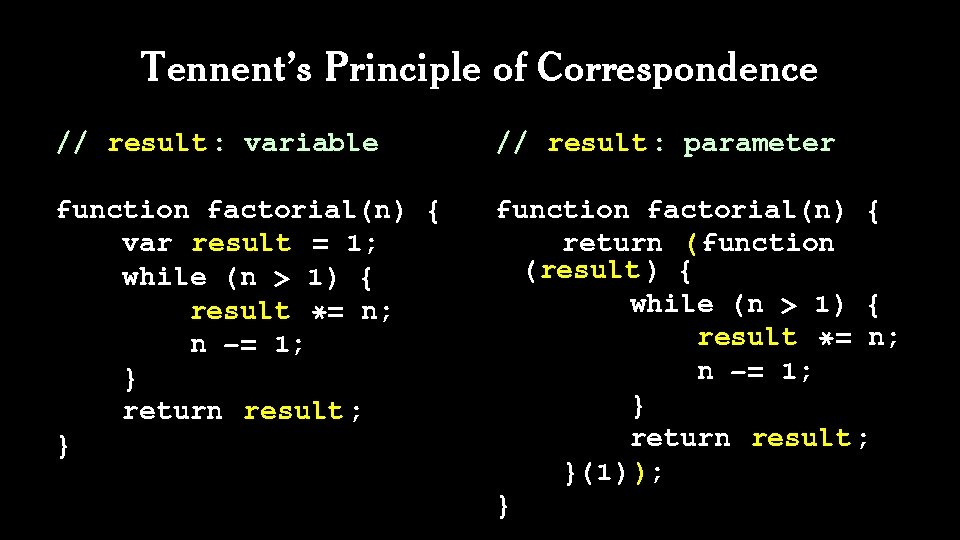Tennent’s Principle of Correspondence // result : variable // result : parameter function factorial(n) { var result = 1; while (n > 1) { result *= n; n -= 1; } return result ; } function factorial(n) { return (function (result ) { while (n > 1) { result *= n; n -= 1; } return result ; }(1)); }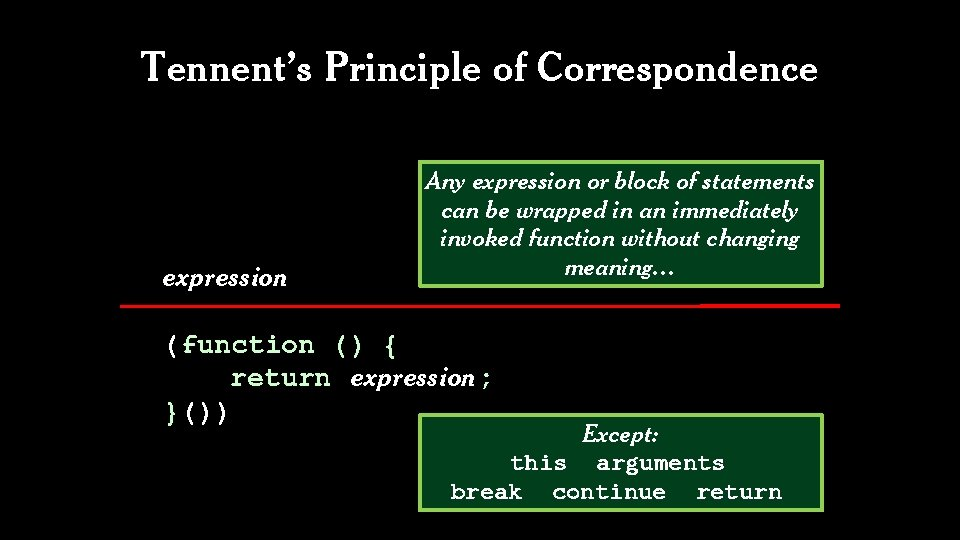Tennent’s Principle of Correspondence expression Any expression or block of statements can be wrapped in an immediately invoked function without changing meaning… (function () { return expression; }()) Except: this arguments break continue returnClosure Lexical Scoping Static Scoping Functions can nest. Functions are values.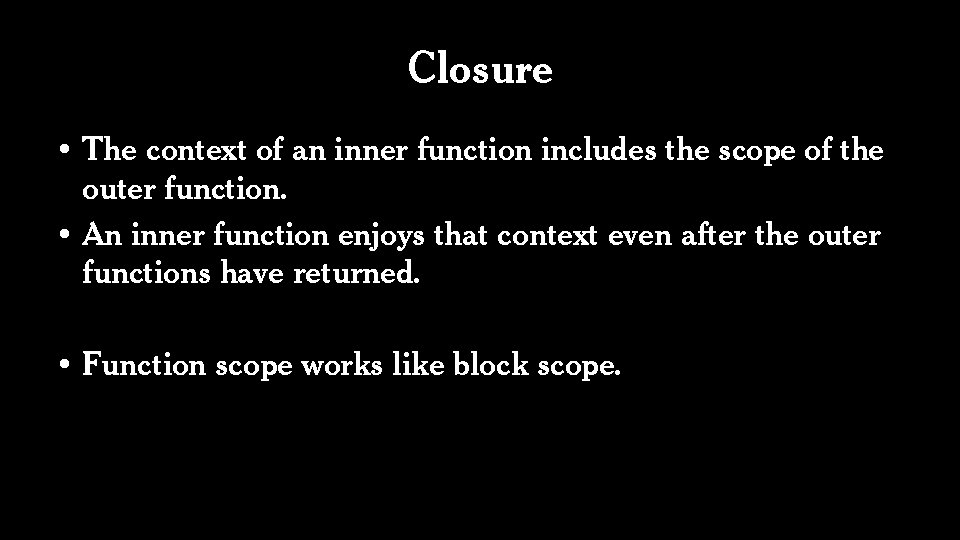Closure • The context of an inner function includes the scope of the outer function. • An inner function enjoys that context even after the outer functions have returned. • Function scope works like block scope.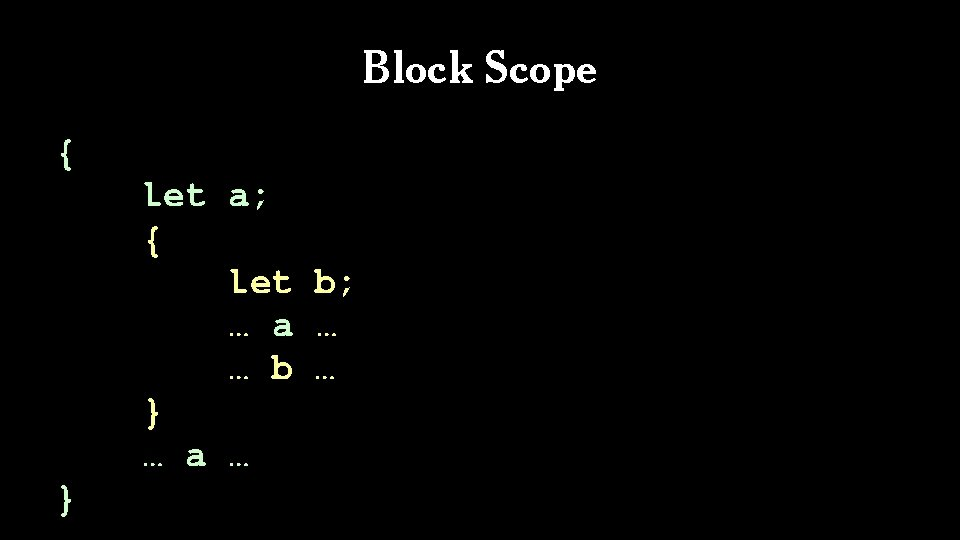Block Scope { let a; { let b; … a … … b … } … a … }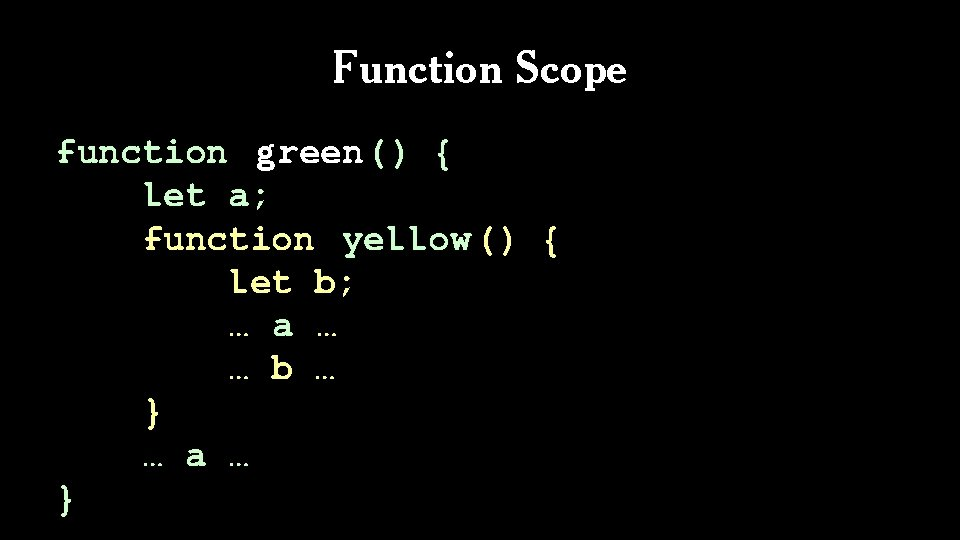Function Scope function green() { let a; function yellow () { let b; … a … … b … } … a … }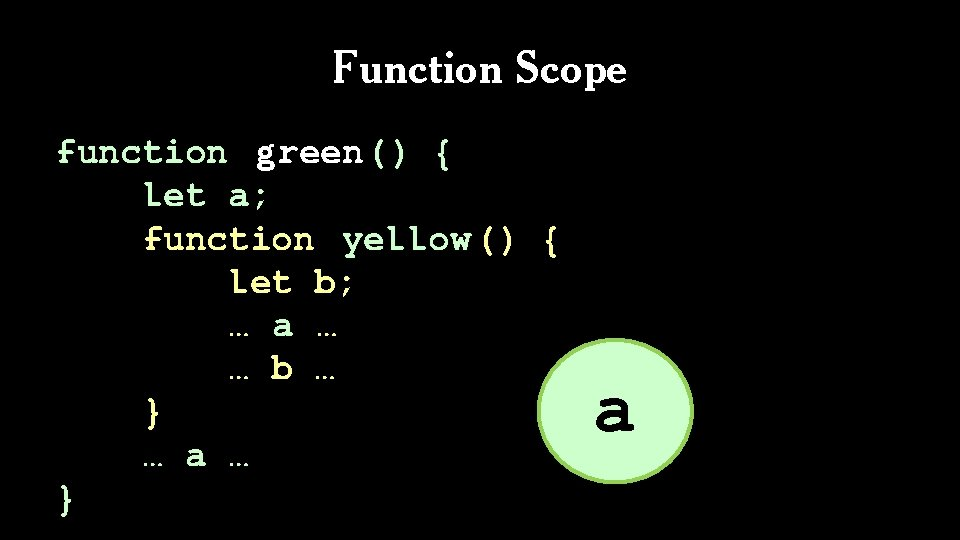Function Scope function green() { let a; function yellow () { let b; … a … … b … } … a … } a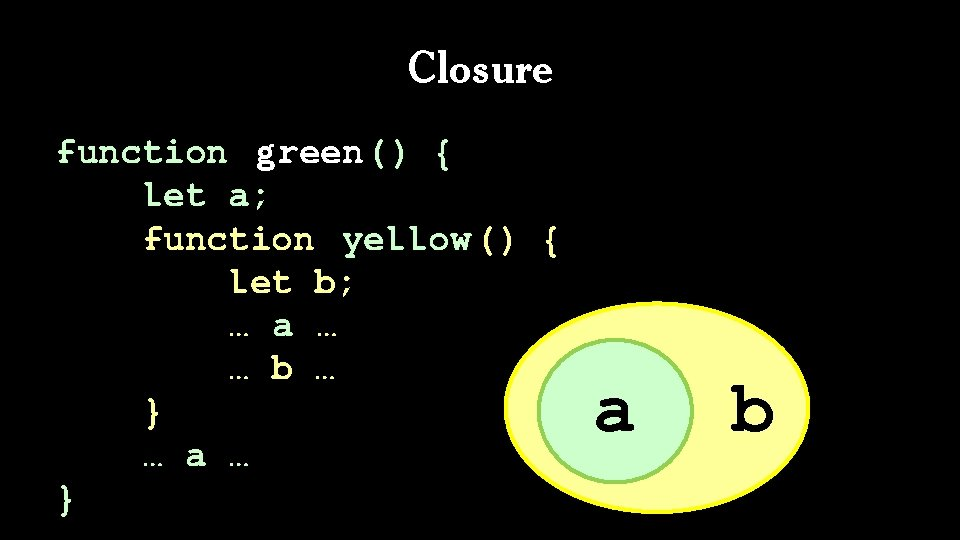Closure function green() { let a; function yellow () { let b; … a … … b … } … a … } a b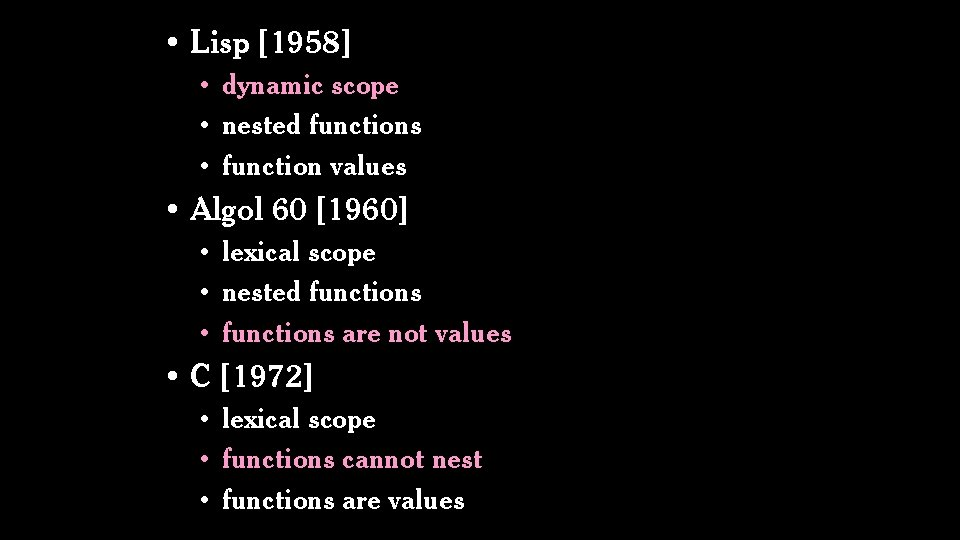• Lisp  • dynamic scope • nested functions • function values • Algol 60  • lexical scope • nested functions • functions are not values • C  • lexical scope • functions cannot nest • functions are values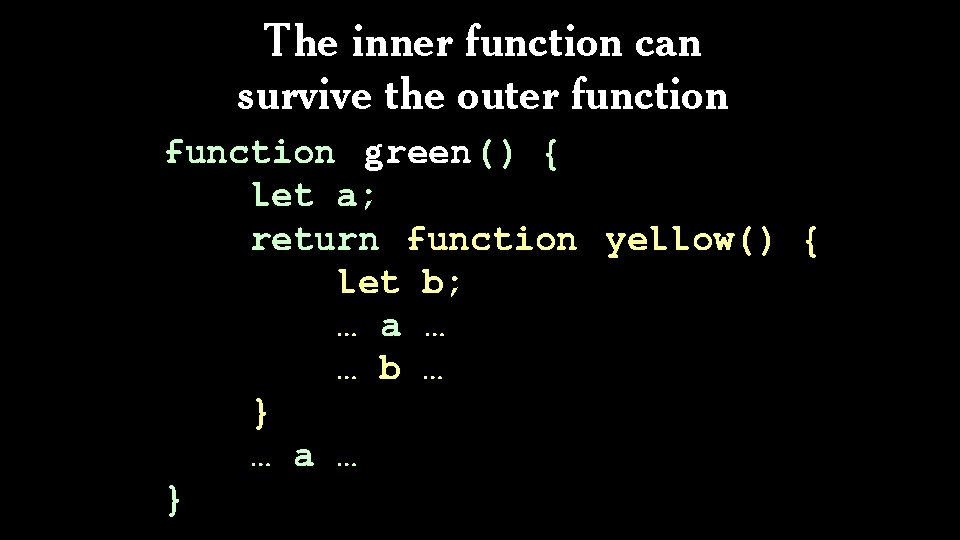The inner function can survive the outer function green() { let a; return function yellow() { let b; … a … … b … } … a … }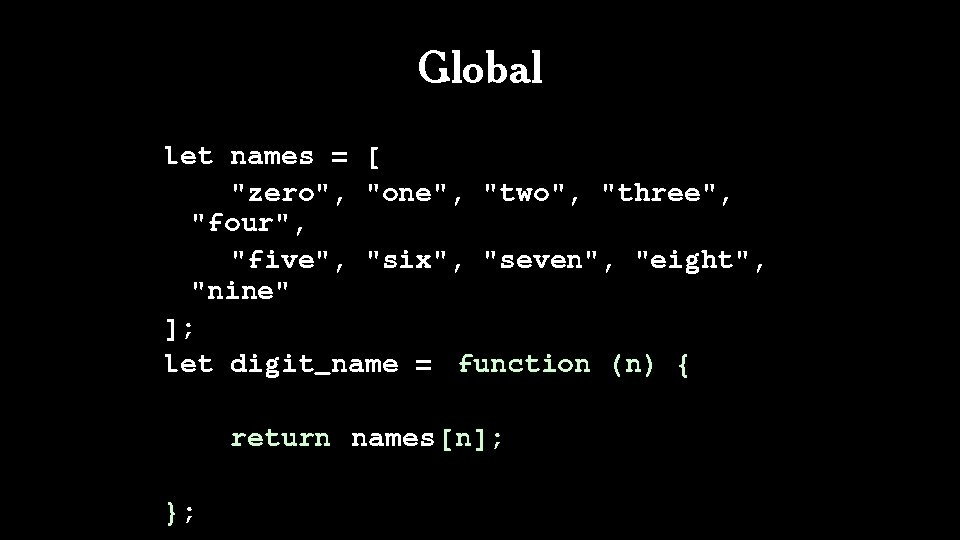Global let names = [ "zero", "one", "two", "three", "four", "five", "six", "seven", "eight", "nine" ]; let digit_name = function (n) { return names [n]; };Slow let digit_name = function (n) { var names = [ "zero", "one", "two", "three", "four", "five", "six", "seven", "eight", "nine" ]; return names[n]; };Closure let digit_name = ( function (n) { var names = [ "zero", "one", "two", "three", "four", "five", "six", "seven", "eight", "nine" ]; return function (n) { return names[n]; }; }());Start Over let names = [ "zero", "one", "two", "three", "four", "five", "six", "seven", "eight", "nine" ]; let digit_name = function (n) { return names [n]; }; alert(digit_name(3)); // "three"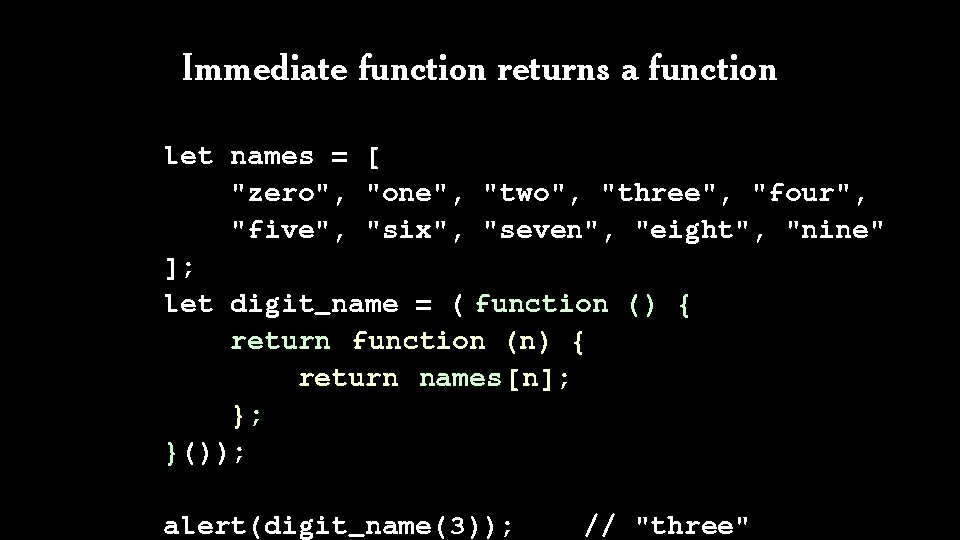Immediate function returns a function let names = [ "zero", "one", "two", "three", "four", "five", "six", "seven", "eight", "nine" ]; let digit_name = ( function () { return function (n) { return names[n]; }; }()); alert(digit_name(3)); // "three"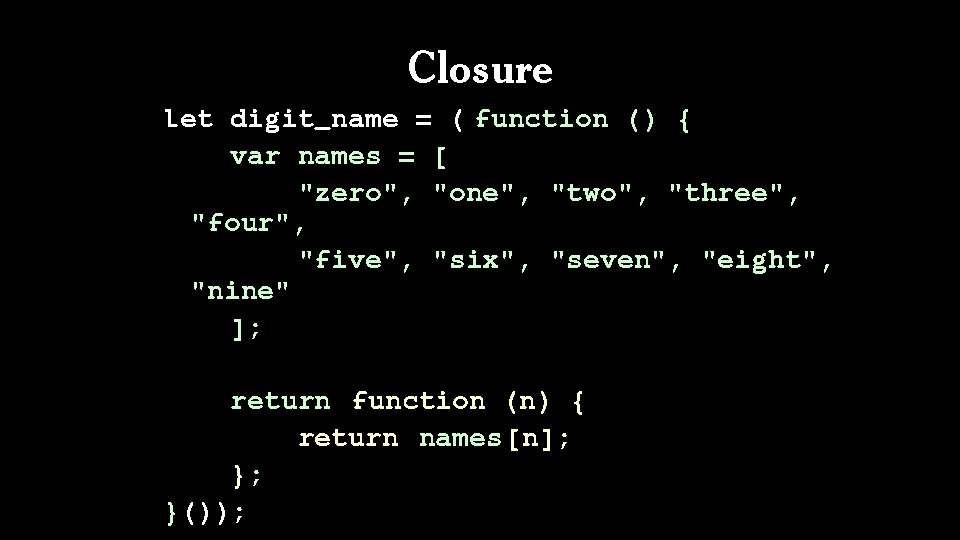Closure let digit_name = ( function () { var names = [ "zero", "one", "two", "three", "four", "five", "six", "seven", "eight", "nine" ]; return function (n) { return names[n]; }; }());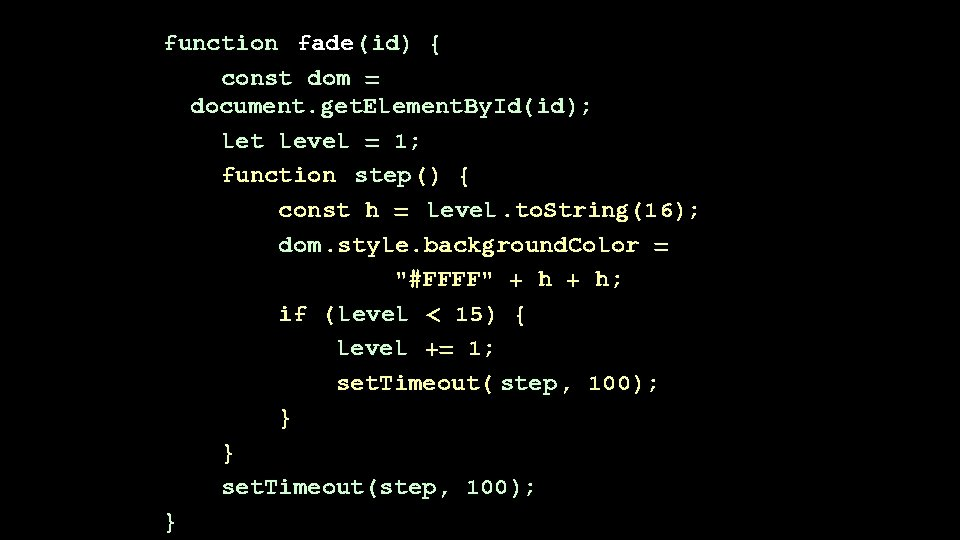function fade(id) { const dom = document. get. Element. By. Id(id); let level = 1; function step() { const h = level. to. String(16); dom. style. background. Color = "#FFFF" + h; if (level < 15) { level += 1; set. Timeout( step, 100); } } set. Timeout(step, 100); }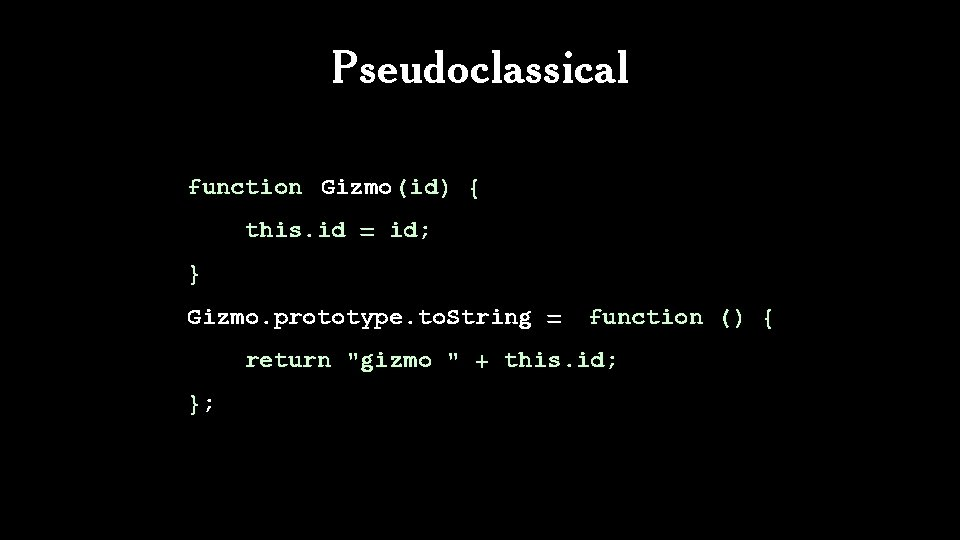Pseudoclassical function Gizmo(id) { this. id = id; } Gizmo. prototype. to. String = function () { return "gizmo " + this. id; };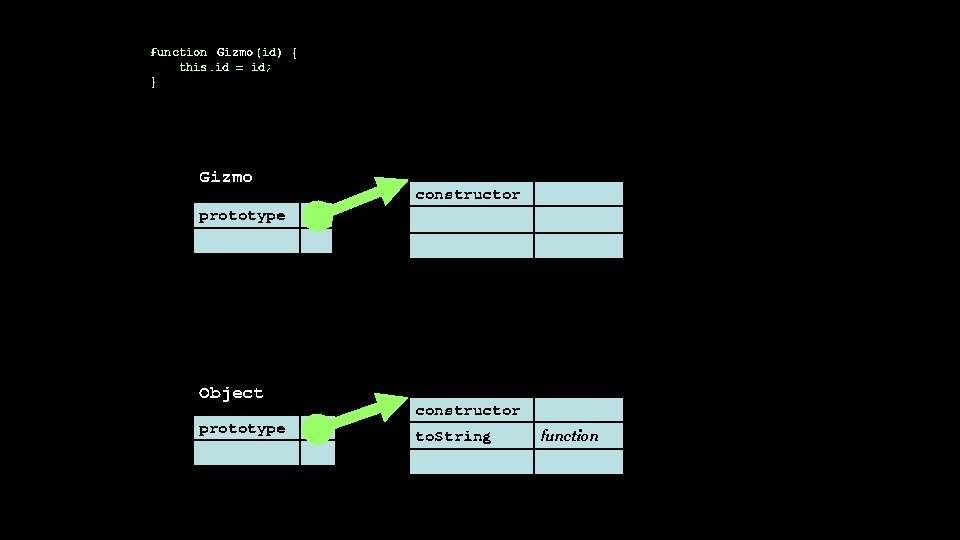function Gizmo (id) { this. id = id; } Gizmo constructor prototype Object prototype constructor to. String functionfunction Gizmo (id) { this. id = id; } Gizmo. prototype. to. String = function () { return "gizmo " + this. id; }; Gizmo prototype Object prototype constructor to. String function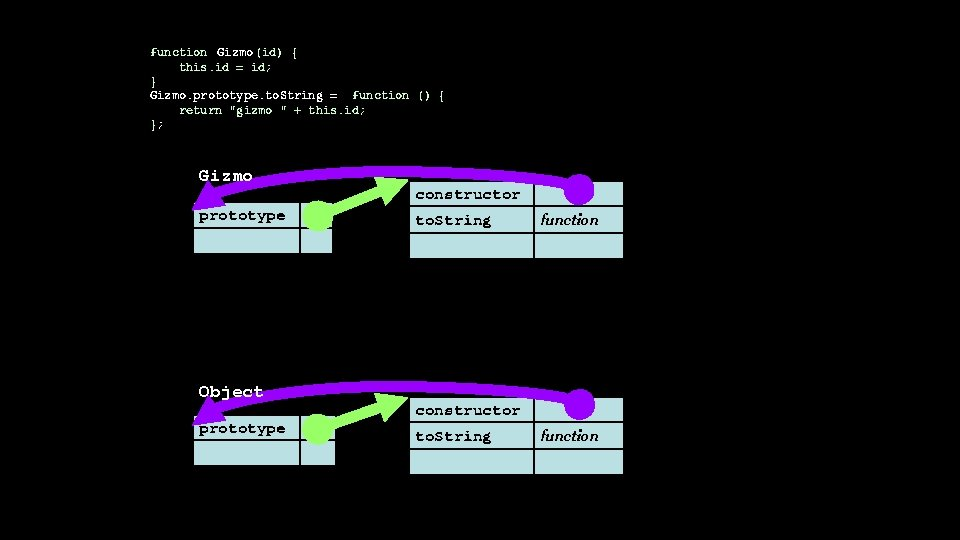function Gizmo (id) { this. id = id; } Gizmo. prototype. to. String = function () { return "gizmo " + this. id; }; Gizmo prototype Object prototype constructor to. String function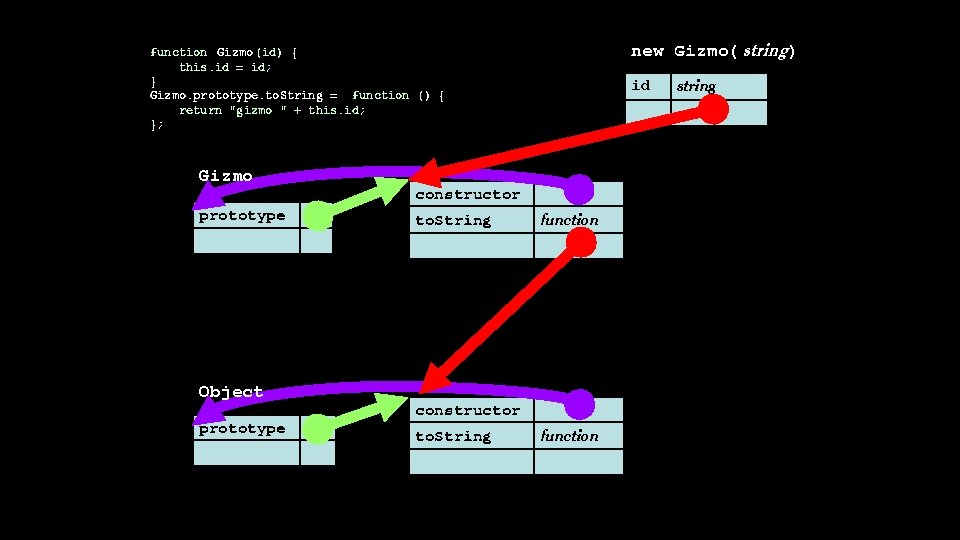new Gizmo( string) function Gizmo (id) { this. id = id; } Gizmo. prototype. to. String = function () { return "gizmo " + this. id; }; Gizmo prototype Object prototype id constructor to. String function string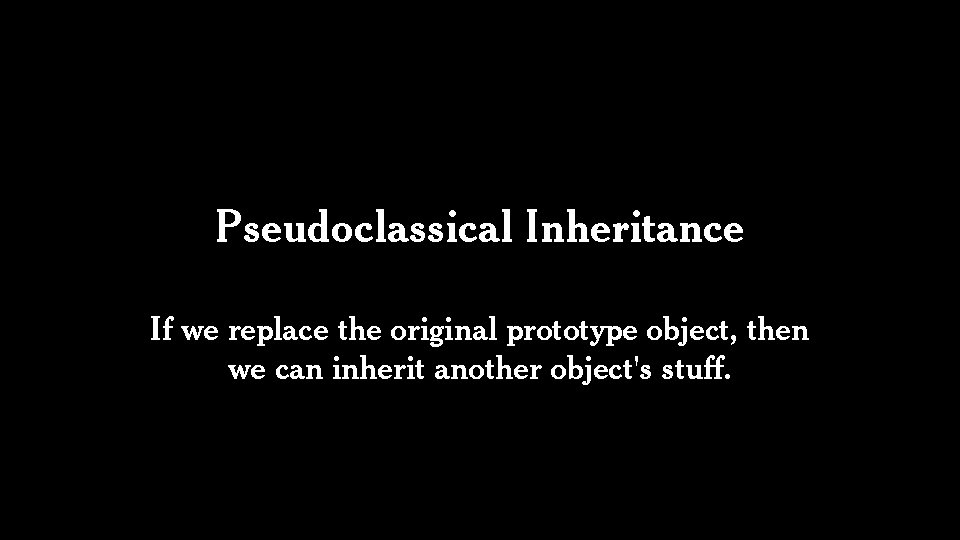Pseudoclassical Inheritance If we replace the original prototype object, then we can inherit another object's stuff.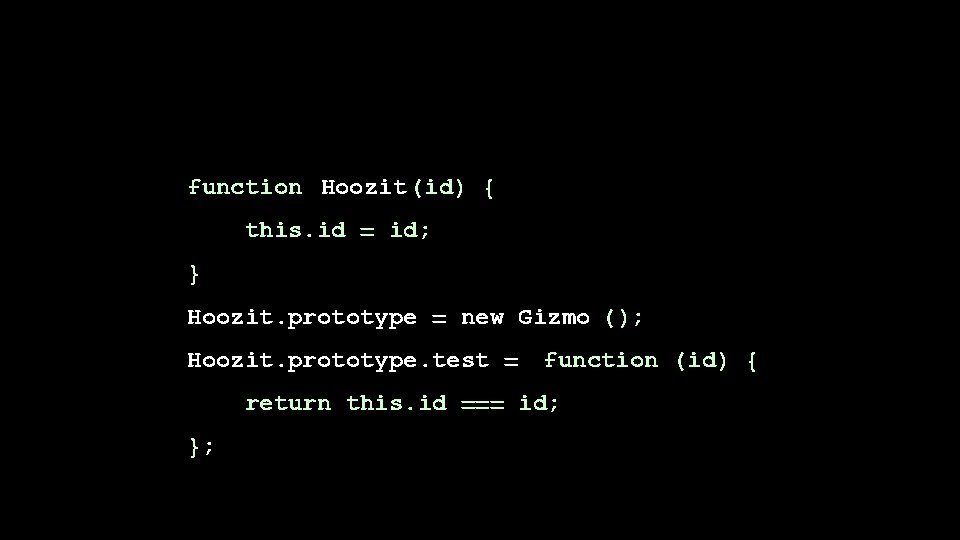function Hoozit (id) { this. id = id; } Hoozit. prototype = new Gizmo (); Hoozit. prototype. test = function (id) { return this. id === id; };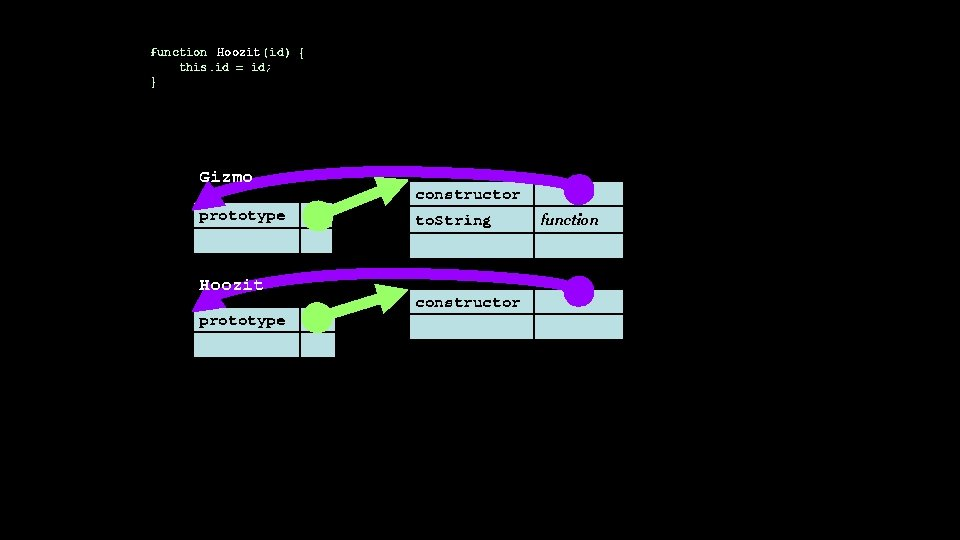function Hoozit (id) { this. id = id; } Gizmo prototype Hoozit prototype constructor to. String constructor function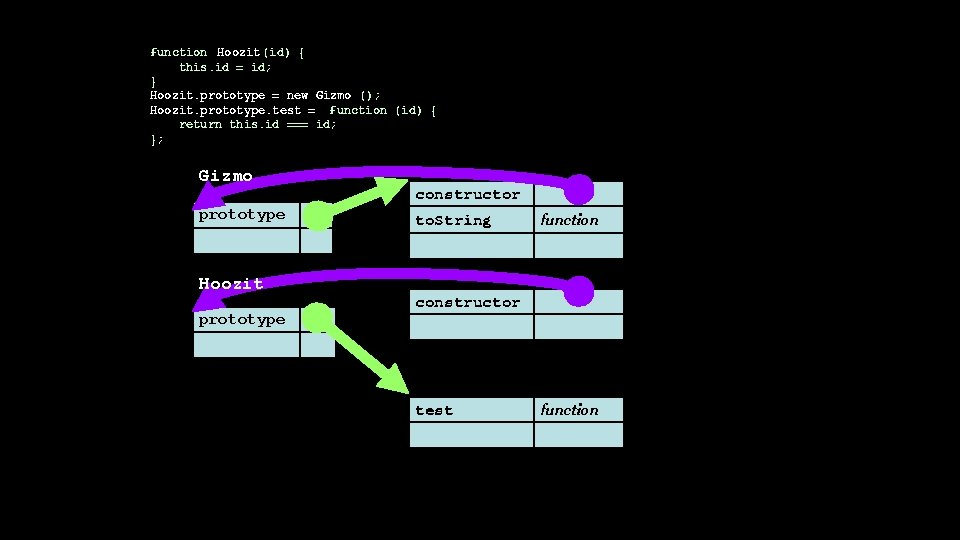function Hoozit (id) { this. id = id; } Hoozit. prototype = new Gizmo (); Hoozit. prototype. test = function (id) { return this. id === id; }; Gizmo prototype Hoozit prototype constructor to. String function constructor test functionfunction Hoozit (id) { this. id = id; } Hoozit. prototype = new Gizmo (); Hoozit. prototype. test = function (id) { return this. id === id; }; Gizmo prototype new Hoozit( string) id constructor to. String function test function Hoozit prototype stringPseudoclassical Inheritance function Gizmo(id) { this. id = id; } Gizmo. prototype. to. String = function () { return "gizmo " + this. id; }; function Hoozit(id) { this. id = id; } Hoozit. prototype = new Gizmo(); Hoozit. prototype. test = function (id) { return this. id === id; };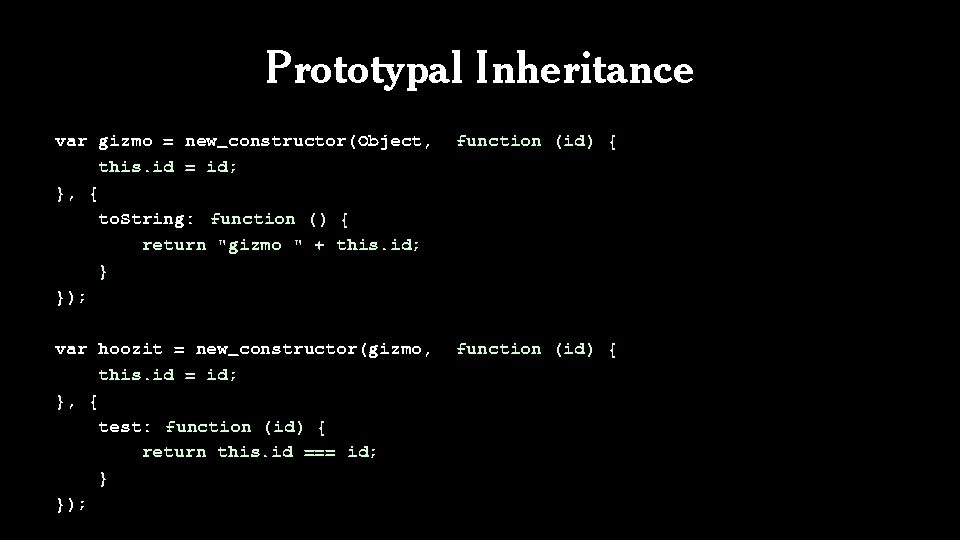Prototypal Inheritance var gizmo = new_constructor(Object, this. id = id; }, { to. String: function () { return "gizmo " + this. id; } }); function (id) { var hoozit = new_constructor(gizmo, this. id = id; }, { test: function (id) { return this. id === id; } }); function (id) {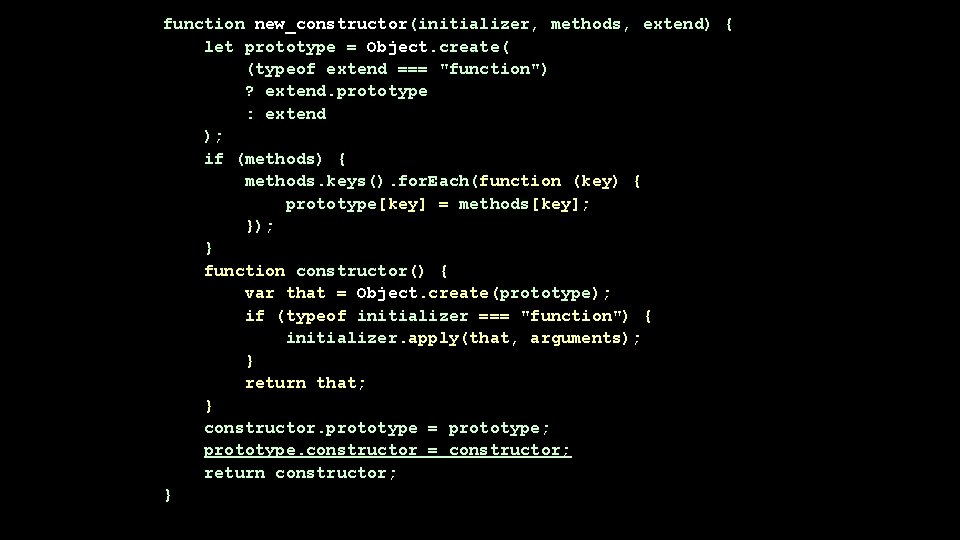function new_constructor(initializer, methods, extend) { let prototype = Object. create( (typeof extend === "function") ? extend. prototype : extend ); if (methods) { methods. keys(). for. Each(function (key) { prototype[key] = methods[key]; }); } function constructor() { var that = Object. create(prototype); if (typeof initializer === "function") { initializer. apply(that, arguments); } return that; } constructor. prototype = prototype; prototype. constructor = constructor; return constructor; }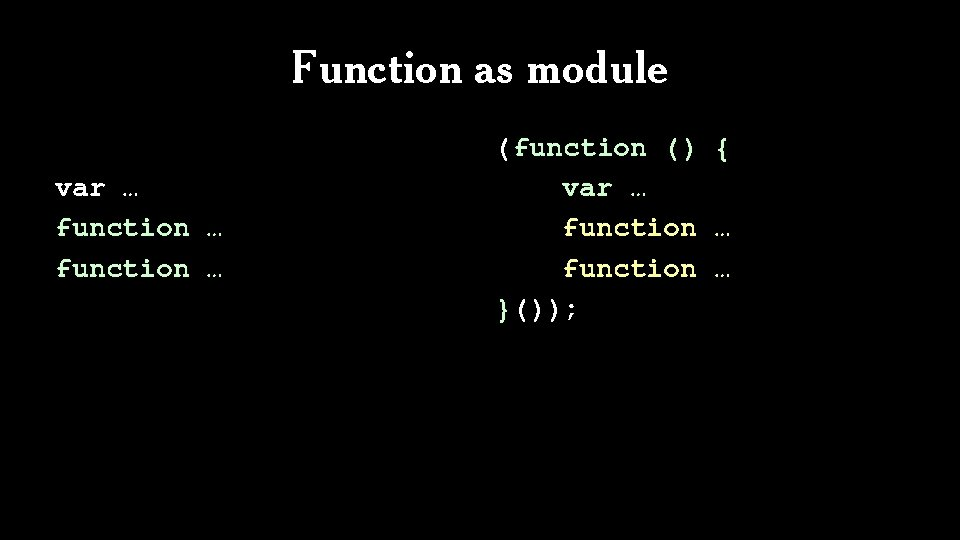Function as module var … function … (function () { var … function … }());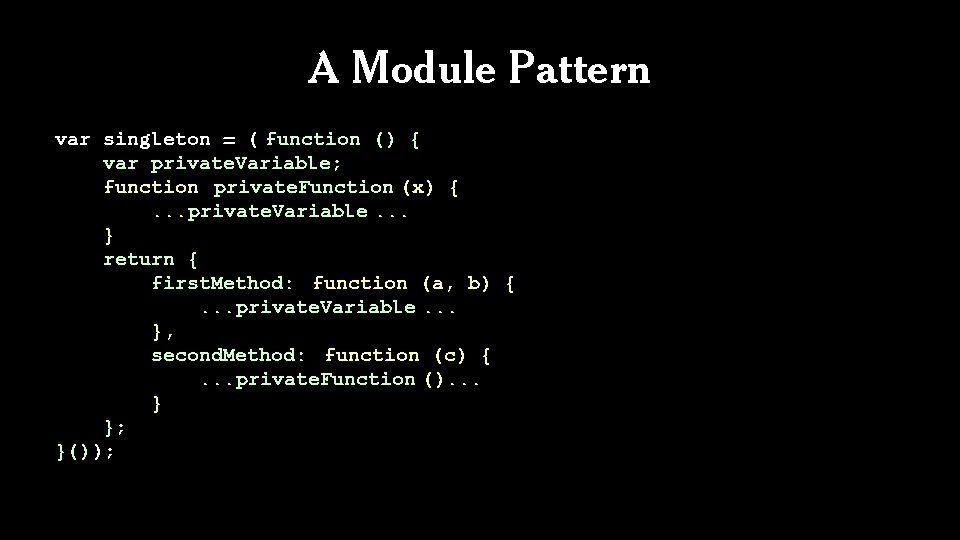A Module Pattern var singleton = ( function () { var private. Variable; function private. Function (x) {. . . private. Variable. . . } return { first. Method: function (a, b) {. . . private. Variable. . . }, second. Method: function (c) {. . . private. Function (). . . } }; }());A Module Pattern (function () { var private. Variable; function private. Function(x) {. . . private. Variable. . . } GLOBAL. methodical = { first. Method: function (a, b) {. . . private. Variable. . . }, second. Method: function (c) {. . . private. Function (). . . } }; }());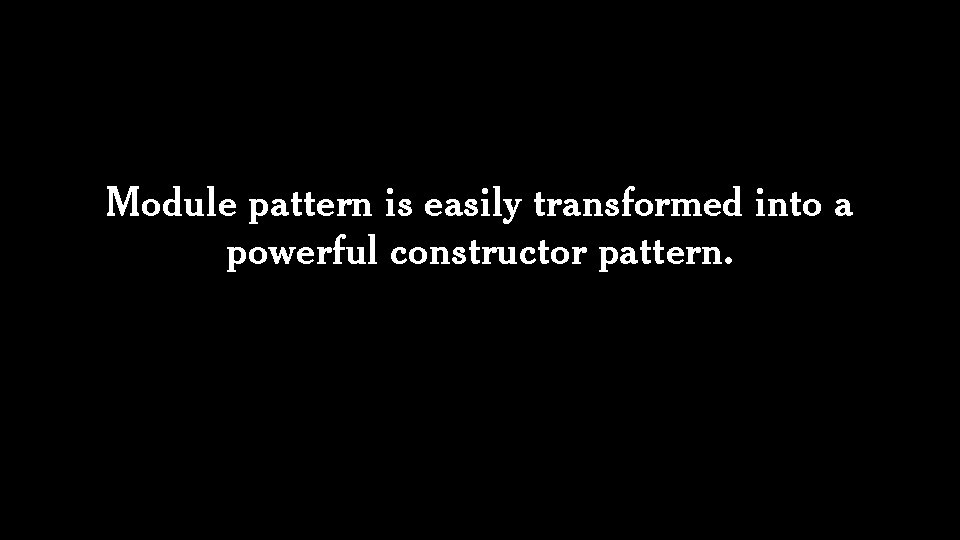Module pattern is easily transformed into a powerful constructor pattern.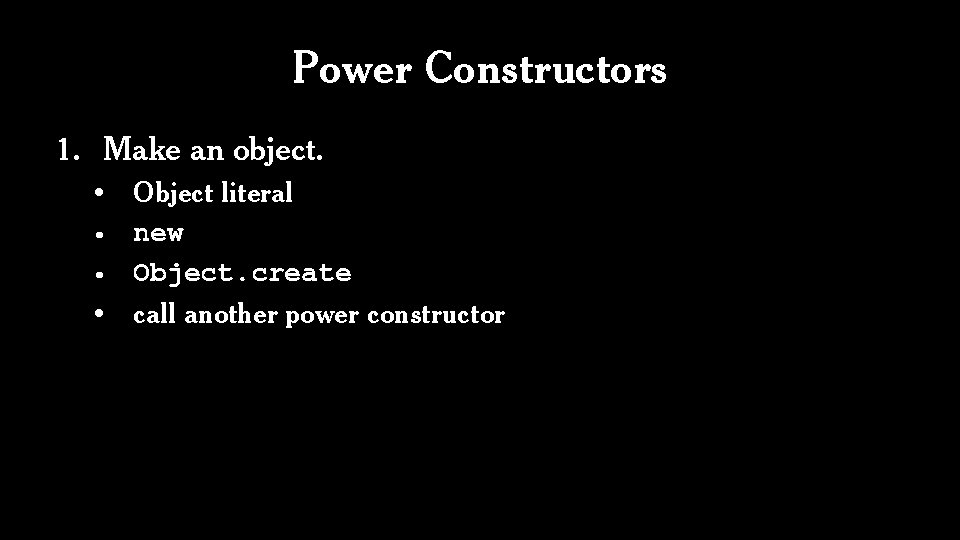Power Constructors 1. Make an object. • • Object literal new Object. create call another power constructorPower Constructors 1. Make an object. • Object literal, new, Object. create, call another power constructor 2. Define some variables and functions. • These become private members.Power Constructors 1. Make an object. • Object literal, new, Object. create, call another power constructor 2. Define some variables and functions. • These become private members. 3. Augment the object with privileged methods.Power Constructors 1. Make an object. • Object literal, new, Object. create, call another power constructor 2. Define some variables and functions. • These become private members. 3. Augment the object with privileged methods. 4. Return the object.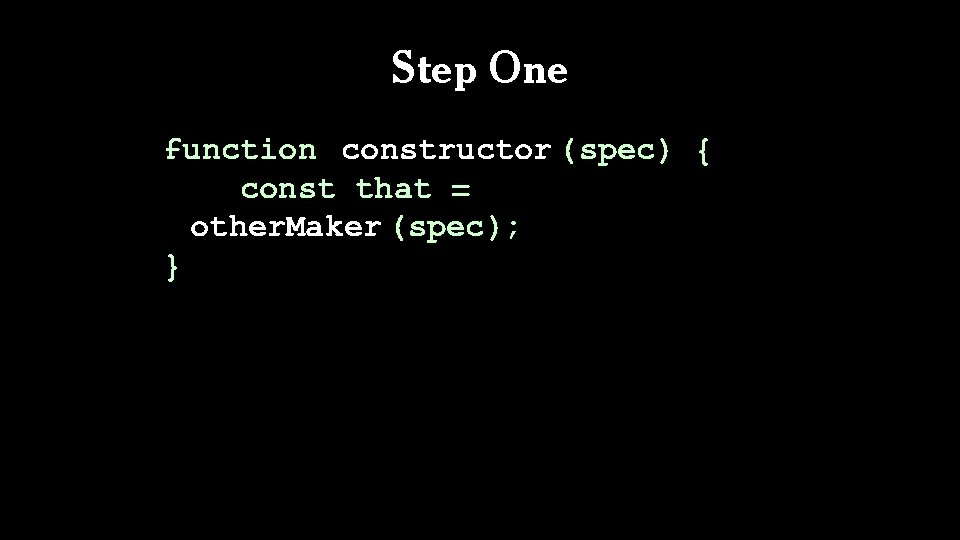Step One function constructor (spec) { const that = other. Maker (spec); }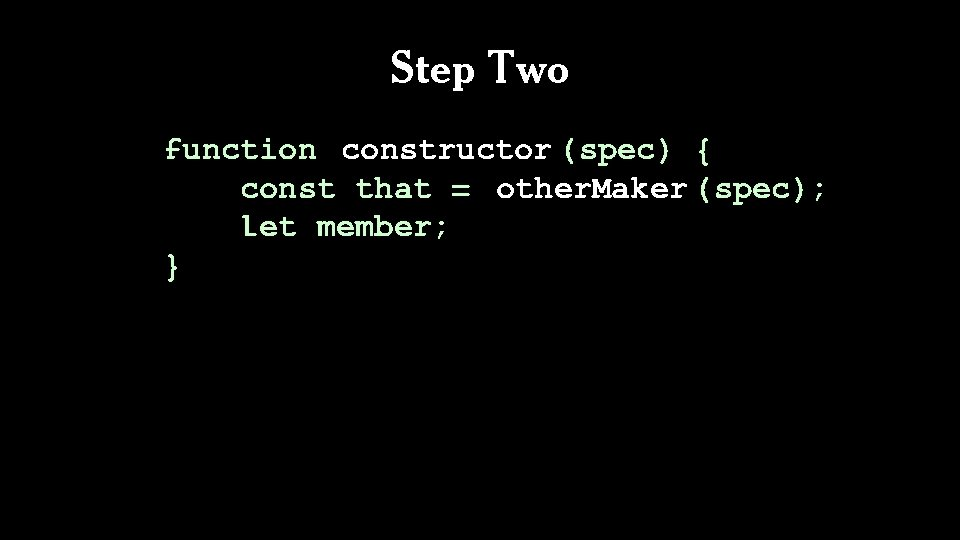Step Two function constructor (spec) { const that = other. Maker (spec); let member; }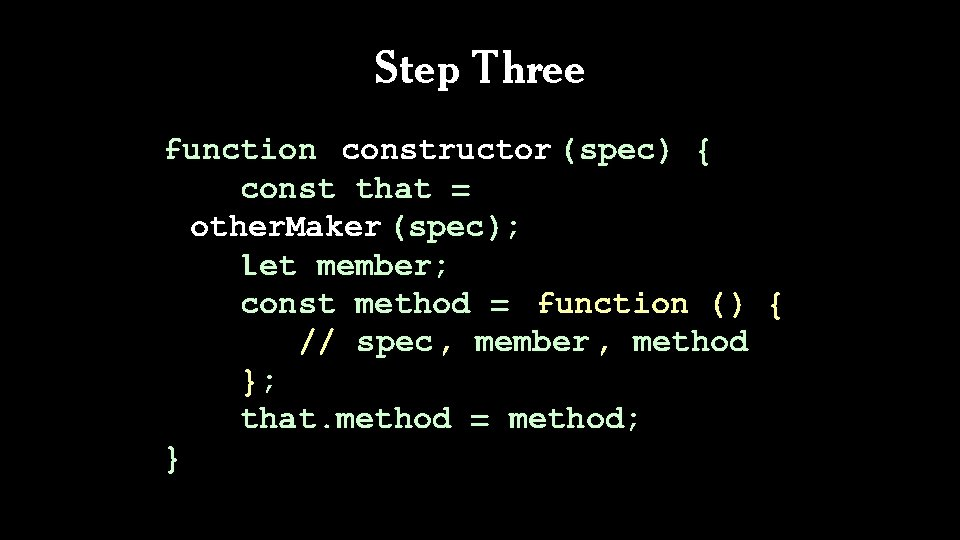Step Three function constructor (spec) { const that = other. Maker (spec); let member; const method = function () { // spec, member , method }; that. method = method; }Step Four function constructor (spec) { const that = other. Maker (spec); let member; const method = function () { // spec, member , method }; that. method = method; return that; }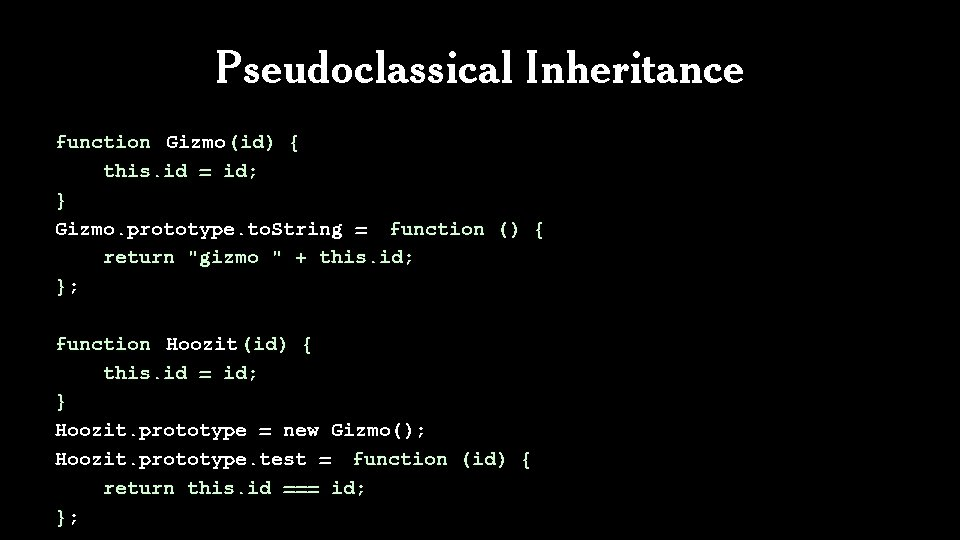Pseudoclassical Inheritance function Gizmo(id) { this. id = id; } Gizmo. prototype. to. String = function () { return "gizmo " + this. id; }; function Hoozit (id) { this. id = id; } Hoozit. prototype = new Gizmo(); Hoozit. prototype. test = function (id) { return this. id === id; };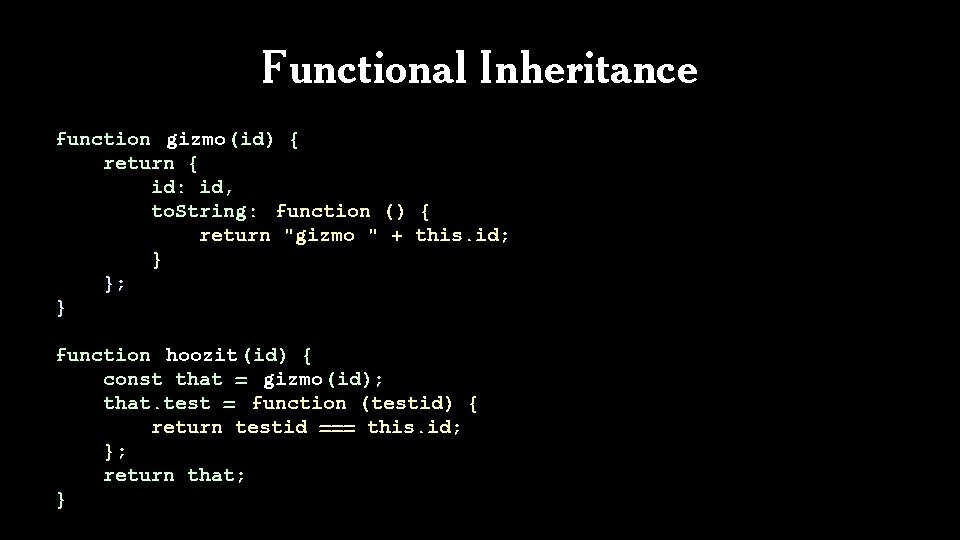Functional Inheritance function gizmo(id) { return { id: id, to. String: function () { return "gizmo " + this. id; } }; } function hoozit (id) { const that = gizmo(id); that. test = function (testid) { return testid === this. id; }; return that; }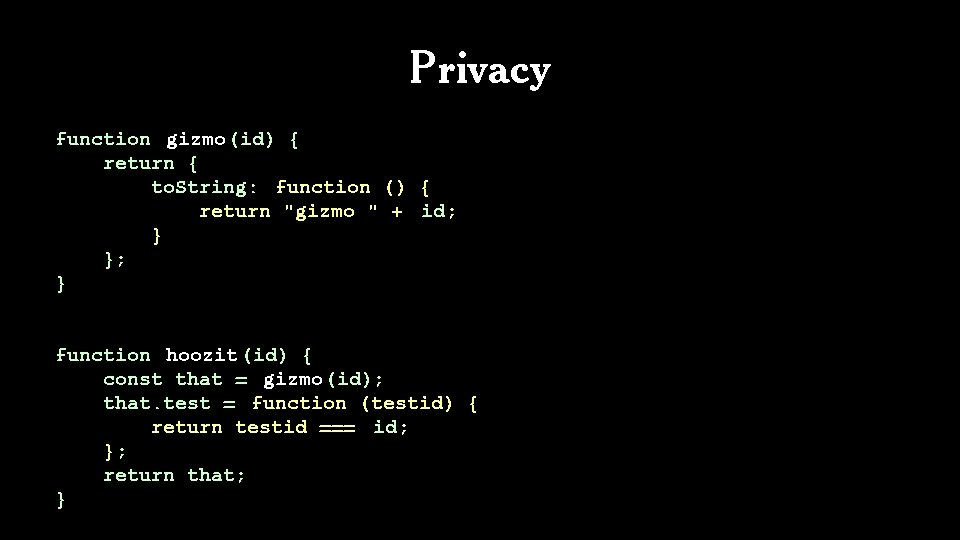Privacy function gizmo(id) { return { to. String: function () { return "gizmo " + id; } }; } function hoozit (id) { const that = gizmo(id); that. test = function (testid) { return testid === id; }; return that; }function Hoozit(id) { this. id = id; } Hoozit. prototype = new Gizmo(); Hoozit. prototype. test = function (id) { return this. id === id; }; function hoozit(id) { var that = gizmo(id); that. test = function (testid) { return testid === id; }; return that; } let my_hoozit = new Hoozit("success"); let test = my_hoozit. test; alert(test("success")); // B O O M !function Hoozit(id) { this. id = id; } Hoozit. prototype = new Gizmo(); Hoozit. prototype. test = function (id) { return this. id === id; }; function hoozit(id) { const that = gizmo(id); that. test = function (testid) { return testid === id; }; return that; } let my_hoozit = hoozit("success"); let test = my_hoozit. test; alert(test("success")); // trueNext: Episode IV The Metamorphosis of Ajax